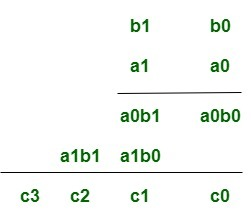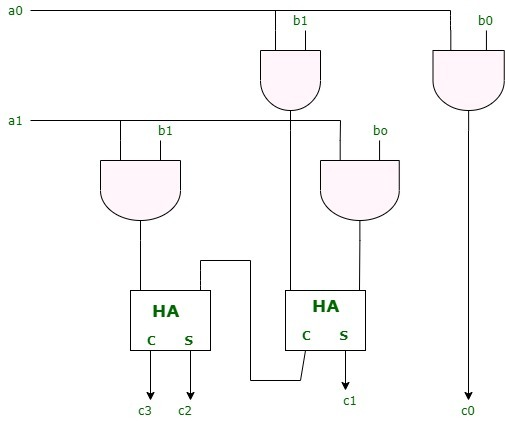# Array Multiplier in Digital Logic

An array multiplier is a digital combinational circuit used for multiplying two binary numbers by employing an array of full adders and half adders. This array is used for the nearly simultaneous addition of the various product terms involved. To form the various product terms, an array of AND gates is used before the Adder array.

Checking the bits of the multiplier one at a time and forming partial products is a sequential operation that requires a sequence of add and shift micro-operations. The multiplication of two binary numbers can be done with one micro-operation by means of a combinational circuit that forms the product bits all at once. This is a fast way of multiplying two numbers since all it takes is the time for the signals to propagate through the gates that form the multiplication array. However, an array multiplier requires a large number of gates, and for this reason it was not economical until the development of integrated circuits.

For implementation of array multiplier with a combinational circuit, consider the multiplication of two 2-bit numbers as shown in figure. The multiplicand bits are b1 and b0, the multiplier bits are a1 and a0, and the product is

`c3c2c1c0`Assuming A = a1a0 and B= b1b0, the various bits of the final product term P can be written as:-
1. P(0)= a0b0
2. P(1)=a1b0 + b1a0
3. P(2) = a1b1 + c1 where c1 is the carry generated during the addition for the P(1) term.
4. P(3) = c2 where c2 is the carry generated during the addition for the P(2) term.

For the above multiplication, an array of four AND gates is required to form the various product terms like a0b0 etc. and then an adder array is required to calculate the sums involving the various product terms and carry combinations mentioned in the above equations in order to get the final Product bits.

1. The first partial product is formed by multiplying a0 by b1, b0. The multiplication of two bits such as a0 and b0 produces a 1 if both bits are 1; otherwise, it produces 0. This is identical to an AND operation and can be implemented with an AND gate.
2. The first partial product is formed by means of two AND gates.
3. The second partial product is formed by multiplying a1 by b1b0 and is shifted one position to the left.
4. The above two partial products are added with two half-adder(HA) circuits. Usually there are more bits in the partial products and it will be necessary to use full-adders to produce the sum.
5. Note that the least significant bit of the product does not have to go through an adder since it is formed by the output of the first AND gate.A combinational circuit binary multiplier with more bits can be constructed in similar fashion. A bit of the multiplier is ANDed with each bit of the multiplicand in as many levels as there are bits in the multiplier. The binary output in each level of AND gates is added in parallel with the partial product of the previous level to form a new partial product. The last level produces the product. For j multiplier bits and k multiplicand we need j*k AND gates and (j-1) k-bit adders to produce a product of j+k bits.

Attention reader! Don’t stop learning now. Get hold of all the important DSA concepts with the DSA Self Paced Course at a student-friendly price and become industry ready.

My Personal Notes arrow_drop_upCheck out this Author's contributed articles.

If you like GeeksforGeeks and would like to contribute, you can also write an article using contribute.geeksforgeeks.org or mail your article to contribute@geeksforgeeks.org. See your article appearing on the GeeksforGeeks main page and help other Geeks.

Please Improve this article if you find anything incorrect by clicking on the "Improve Article" button below.

2

Please write to us at contribute@geeksforgeeks.org to report any issue with the above content.